# Class 11 Maths NCERT Solutions for Chapter 11 Conic Sections Miscellaneous Exercise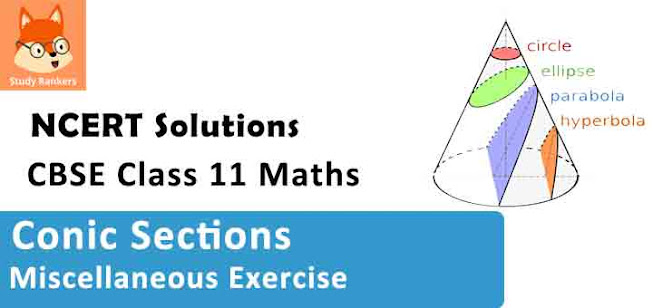### Conic Sections Miscellaneous Exercise Solutions

1. If a parabolic reflector is 20 cm in diameter and 5 cm deep, find the focus.

Solution

The origin of the coordinate plane is taken at the vertex of the parabolic reflector in such a way that the axis of the reflector is along the positive x-axis.
This can be diagrammatically represented as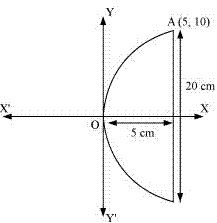The equation of the parabola is of the form y2 = 4ax (as it is opening to the right).
Since the parabola passes through point A (10, 5), 102 = 4a(5)
⇒ 100 = 20a
⇒ a = 100/20 = 5
Therefore, the focus of the parabola is (a, 0) = (5, 0), which is the mid-point of the diameter.
Hence, the focus of the reflector is at the mid-point of the diameter.

2. An arch is in the form of a parabola with its axis vertical. The arch is 10 m high and 5 m wide at the base. How wide is it 2 m from the vertex of the parabola?

Solution

The origin of the coordinate plane is taken at the vertex of the arch in such a way that its vertical axis is along the negative y-axis.
This can be diagrammatically represented as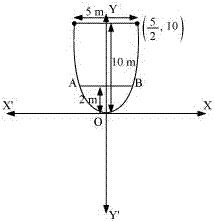The equation of the parabola is of the form x2 = −4ay (as it is opening downwards).
It can be clearly seen that the parabola passes through point (5/2,−10).
(5/2)2 = 4a (10)
⇒ 4a = 25/(4 × 10) = 5/8
Therefore, the arch is in the form of a parabola whose equation is x2 = (-5/8)y .
When y = -2, x2 = (-5/8) (-2)
⇒ x2 = 5/4
⇒ x = √5/2
∴ AB = 2× (√5/2) m = √5 m = 2.23 m (approx.)
Hence , when the arch is  2 m from the vertex of the parabola, its width is approximately 2.23m.

3. The cable of a uniformly loaded suspension bridge hangs in the form of a parabola. The roadway which is horizontal and 100 m long is supported by vertical wires attached to the cable, the longest wire being 30 m and the shortest being 6 m. Find the length of a supporting wire attached to the roadway 18 m from the middle.

Solution

The vertex is at the lowest point of the cable. The origin of the coordinate plane is taken as the vertex of the parabola, while its vertical axis is taken along the positive y-axis. This can be diagrammatically represented as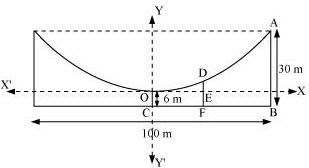Here, AB and OC are the longest and the shortest wires, respectively, attached to the cable.
DF is the supporting wire attached to the roadway, 18 m from the middle.
Here, AB = 30 m, OC = 6 m, and BC = 100/2 = 50 m.
The equation of the parabola is of the form x2 = 4ay (as it is opening upwards).
The coordinates of point A are (50, 30 – 6) = (50, 24).
Since A (50, 24) is a point on the parabola,
(50)2 = 4a(24)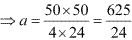Equation of the parabola, x2 = 4 × 625/24 × y or 6x2 = 625y
The x - coordinate of point D is 18.
Hence, at x = 18,
6(18)2 = 625y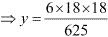⇒ y = 3.11 (approx)
DE = 3.11 m
DF = DE + EF = 3.11m + 6m = 9.11 m
Thus, the length of the supporting wire attached to the roadway 18 m from the middle is approximately 9.11 m.

4. An arch is in the form of a semi-ellipse. It is 8 m wide and 2 m high at the centre. Find the height of the arch at a point 1.5 m from one end.

Solution

Since the height and width of the arc from the centre is 2 m and 8 m respectively, it is clear that the length of the major axis is 8 m, while the length of the semi-minor axis is 2 m.
The origin of the coordinate plane is taken as the centre of the ellipse, while the major axis is taken along the x-axis. Hence, the semi-ellipse can be diagrammatically represented as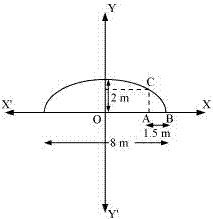The equation of the semi - ellipse will be of the form x2/a2 + y2/b2 = 1, y ≥ 0 , where a is the semi- major axis
Accordingly, 2a = 8
⇒ a = 4
b = 2
Therefore, the equation of the semi-ellipse is x2/16 + y2 /4 = 1, y ≥ 0 ...(1)
Let A be a point on the major axis such that AB = 1.5 m.
Draw AC⊥ OB.
OA = (4 – 1.5) m = 2.5 m
The x-coordinate of point C is 2.5.
On substituting the value of x with 2.5 in equation (1), we obtain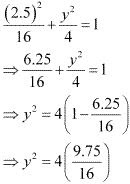⇒ y2 = 2.4375
⇒ y = 1.56 (approx.)
DAC = 1.56 m
Thus, the height of the arch at a point 1.5 m from one end is approximately 1.56 m.

5. A rod of length 12 cm moves with its ends always touching the coordinate axes. Determine the equation of the locus of a point P on the rod, which is 3 cm from the end in contact with the x-axis.

Solution

Let AB be the rod making an angle θ with OX and P (x, y) be the point on it such that AP = 3 cm.
Then, PB = AB – AP = (12 – 3) cm = 9 cm [AB = 12 cm]
From P, draw PQ ⊥OY and PR ⊥ OX.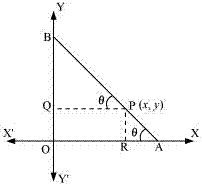In Δ PBQ, cos θ = PQ/PB = x/9
In Δ PRA, sin θ = PR/PA = y/3
Since, sin2 θ + cos2 θ = 1,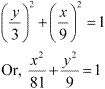Thus, the equation of the locus of point P on the rod is x2/81 + y2/9 = 1.

6. Find the area of the triangle formed by the lines joining the vertex of the parabola x2 = 12y to the ends of its latus rectum.

Solution

The given parabola is x2 = 12y.
On comparing this equation with x2 = 4ay, we obtain 4a = 12 ⇒ a = 3
∴The coordinates of foci are S (0, a) = S (0, 3)
Let AB be the latus rectum of the given parabola.
The given parabola can be roughly drawn as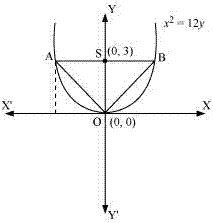At y = 3, x2 = 12 (3) ⇒ x2 = 36 ⇒ x = ±6
∴The coordinates of A are (–6, 3), while the coordinates of B are (6, 3).
Therefore, the vertices of ΔOAB are O (0, 0), A (–6, 3), and B (6, 3).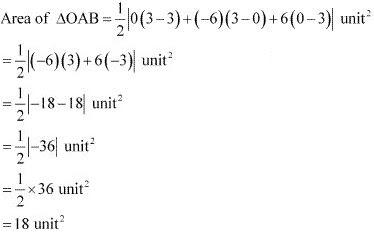Thus, the required area of the triangle is 18 unit2.

7. A man running a racecourse notes that the sum of the distances from the two flag posts form him is always 10 m and the distance between the flag posts is 8 m. find the equation of the posts traced by the man.

Solution

Let A and B be the positions of the two flag posts and P(x, y) be the position of the man. Accordingly, PA + PB = 10.
We know that if a point moves in a plane in such a way that the sum of its distances from two fixed points is constant, then the path is an ellipse and this constant value is equal to the length of the major axis of the ellipse.
Therefore, the path described by the man is an ellipse where the length of the major axis is 10 m, while points A and B are the foci.
Taking the origin of the coordinate plane as the centre of the ellipse, while taking the major axis along the x-axis, the ellipse can be diagrammatically represented as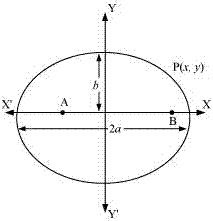The equation of the ellipse will be of the form x2/a2 + y2/b2 = 1, where a is the semi - major axis
Accordingly, 2a = 10
a = 5
Distance between the foci (2c) = 8
c = 4
On using the relation c = √(a2 - b2) , we obtain
4 = √(25 - b2)
⇒ 16 = 25 - b2
⇒ b2 = 25 - 16 = 9
⇒ b = 3
Thus, the equation of the path traced by the man is x2/25 + y2/9 = 1.

8. An equilateral triangle is inscribed in the parabola y2 = 4 ax, where one vertex is at the vertex of the parabola. Find the length of the side of the triangle.

Solution

Let OAB be the equilateral triangle inscribed in parabola y2 = 4ax.
Let AB intersect the x-axis at point C.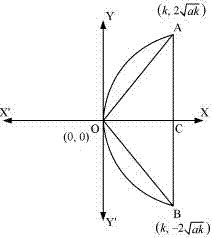Let OC = k
From the equation of the given parabola, we have y2 = 4ak ⇒ y = ± 2√ak
∴ The respective coordinates of points A and B are (k, 2√ak), and (k, -2√ak)
AB = CA + CB = 2√ak + 2√ak = 4√ak
Since OAB is an equilateral triangle, OA2 = AB2 .
∴ k2 + (2√ak)2 = (4√ak)2
⇒ k2 + 4ak = 16ak
⇒ k2 = 12ak
⇒ k = 12a
∴ AB = 4√ak = 4√a × 12a = 4√12a2 = 8√3a
Thus, the side of the equilateral triangle inscribed in parabola y2 = 4 ax is 8√3a.